## Taking apart quick table calculations in Tableau

by Daniel Watt

Taking something apart and putting it back together is a great way to understand how something how something works. This is true for engines, computers and quick table calculations in Tableau. When I started out using Tableau I would use the quick table calculations and change how they were computed to get something that looked right without fully understanding how they worked. But for more complex charts it helps to understand the underlying functions.  So we’re going to take a look at my most commonly used quick table calculations: Running total, difference, percent difference, percent of total and moving average. Each table calculation can be configured to work across rows, columns, the entire table and various combinations of fields. But I’m not going to cover that here, rather just have a look at the underlying functions.

## Running total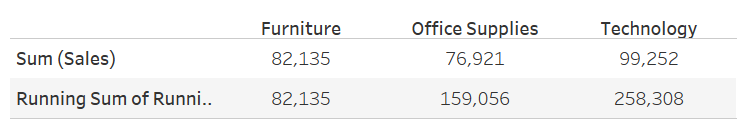Running total is adding each column to the total of all the preceding columns (to the left). So in this example, the running sum of sales for office supplies = sum(sales) for furniture + sum(sales) for office supplies.  The RT of technology equals the total for all three categories. The values you get back for each category depend on the sort order and how the table calculation is computed.

RUNNING_SUM(SUM([Sales]))

The calculated field behind running total uses the RUNNING_SUM function. If there are null values in your table, this is not an issue with this function as it just ignores them and continues the running total at the next non-null value. You could edit this calculation by putting an if statement within the brackets:

eg. RUNNING_SUM(if sum([Sales]) >100 then sum([Sales]) else 0 end)

or you could have a percent of total sales within the brackets (a secondary table calculation):

eg. RUNNING_SUM(SUM([Sales])) / TOTAL(SUM([Sales]))

## Difference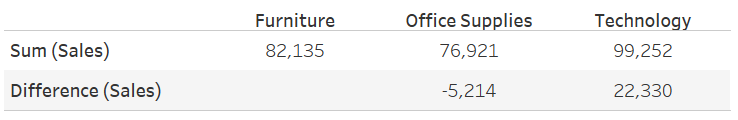Difference subtracts the current column from the previous column (depending how the calculation is computed). The default quick table calculation is as follows:

ZN(SUM([Sales])) – LOOKUP(ZN(SUM([Sales])), -1)

Difference is a bit more interesting as it contains ZN and LOOKUP in the calculation. The ZN stands for Zero Null and turns Null values into Zero. If you did not have the ZNs in the calculation then the difference would be calculated as null if either the current or previous value are null. This may be something that you need to play with inside the calculation, depending on what null means within your data.

LOOKUP is not to be confused with how the lookup functions work in excel.  LOOKUP in Tableau looks at a relative position in the table, eg. the previous row: LOOKUP(Sum([Sales]),-1)  or   two rows ahead:  LOOKUP(SUM([Sales]),2).

## Percent Difference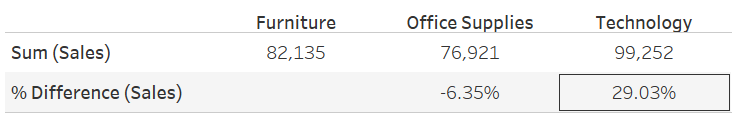Percent difference takes the calculation from Difference (current row minus previous row) and divides by the absolute value of the previous row. The default quick table calculation is as follows:

(ZN(SUM([Sales])) – LOOKUP(ZN(SUM([Sales])), -1)) / ABS(LOOKUP(ZN(SUM([Sales])), -1))

ABS() turns a number into an absolute value. This means that the output number is positive whether the input number is positive or negative. This is required when calculated the percentage difference between negative values. Dividing a negative by a negative will give a positive which will be not show the correct percentage difference. In the chart below I have compared the results you would get when running the calculation with or without the ABS function included: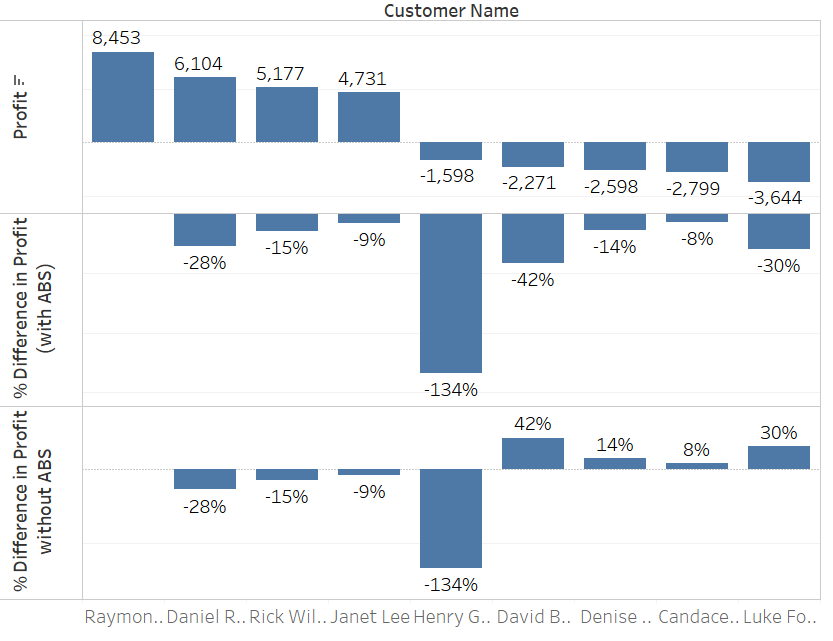## Percent of Total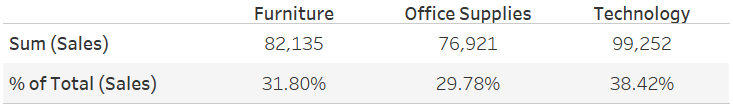Percent of total can be computed to calculate across rows, columns, panes, the whole table or specific dimensions.

SUM([Sales]) / TOTAL(SUM([Sales]))

The TOTAL function totals all the values in a row, column, cell, table or specific dimension.

## Moving average

When you add moving average as a quick calculation, the default is to take the average of the current value and the two previous values in the row (or however the table calculation is computed).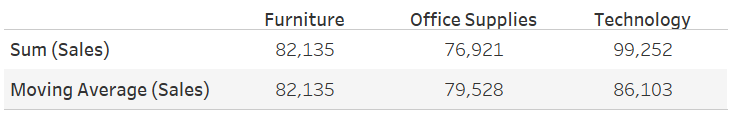The default quick table calculation is as follows:

WINDOW_AVG(SUM([Sales]), -2, 0)

WINDOW_AVG(SUM([Sales]), [start], [end])

The WINDOW_AVG function takes the average of all cells from the start reference to the end reference. In this case it is taking the average of the values between column -2 (2 columns previous) and column 0 (the current column). You could edit this calculation by replacing with different start and end numbers eg. 6,0 to get a 7 day moving average if your table is aggregated by days.  You could also replace the start and end references with other functions such as first() and last(). Last gives you the number of rows from the current row to the last row in the partition, whereas first gives you the number of rows from the current row to the first row. Using first in with window_avg or lookup means that any cell will be referencing the first cell in the partition, whereas using last will always reference the last cell in a partition.Daniel Watt

Fri 06 Jan 2017

Thu 05 Jan 2017

Wed 04 Jan 2017

Tue 13 Dec 2016